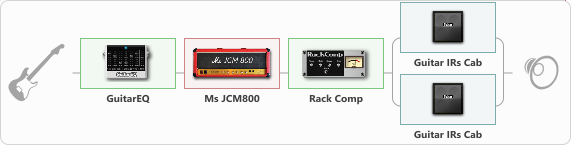# Late 60's Marshall...

Discussion in 'ToneLib-GFX presets' started by Jean C.Guion, Jul 30, 2020.

1. Late 60's Marshall...

Preset name: late 60's Marshall....

using the rack compressor to emalulate a little more power amp compression,,,and a couple Fender 1x12"s miced at different points to give a ballsy but still janggly live sound...very reminescent of jimi hendrix's studio sound...

Effects chain:Effect: "GuitarEQ" (Dynamics / Filter), active - "yes"
{
"160 Hz" = 2
"400 Hz" = 2
"800 Hz" = 7
"1.6 kHz" = 7
"3.2 kHz" = -11
"6.4 kHz" = -15
"12 kHz" = 0
"Level (dB)" = 3
}

Effect: "Ms JCM800" (Amp simulators), active - "yes"
{
"Gain" = 69
"Bass" = 64
"Middle" = 43
"Treble" = 56
"Presence" = 2
"Master" = 67
"Level (dB)" = -1
}

Effect: "Rack Comp" (Dynamics / Filter), active - "yes"
{
"Threshold (dB)" = -16
"Ratio" = 3
"Attack" = Slow
"Release (ms)" = 98
"Knee" = 29
"Level (dB)" = 0
}

Effect: "Splitter" (Dynamics / Filter), active - "yes"
{
"A-Bypass" = Off
"A-Pan" = 0
"A-Level" = 55
"B-Bypass" = Off
"B-Pan" = 0
"B-Level" = 55

'A' branch:
{

Effect: "Guitar IRs Cab" (Cabinets), active - "yes"
{
"Model" = Fender Deluxe Rev (1x12")
"Mic Position" = Middle
"Mic Distance" = Near
"Low Cut (Hz)" = 83
"Hi Cut (kHz)" = 5.0
"Mix" = 100
"Level (dB)" = 1
}
}
'B' branch:
{

Effect: "Guitar IRs Cab" (Cabinets), active - "yes"
{
"Model" = Fender Deluxe Rev (1x12")
"Mic Position" = Middle
"Mic Distance" = Far
"Low Cut (Hz)" = 65
"Hi Cut (kHz)" = 14.0
"Mix" = 100
"Level (dB)" = 5
}
}
}

Note: You will need to download and install the ToneLib-GFX software to use the preset.

File size:
920 bytes
Views:
2,835